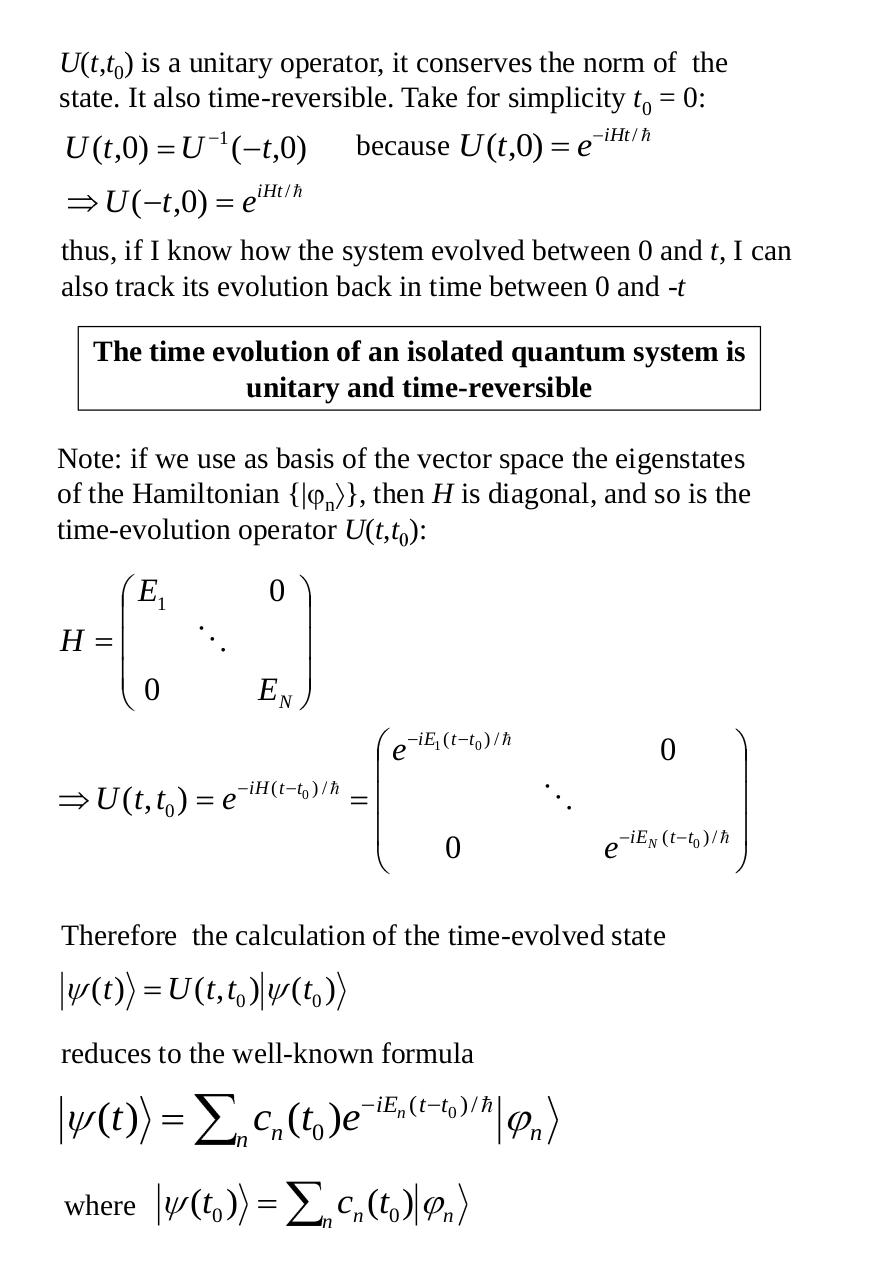# ELEC9705 lecture 05 Operators, Coupling, Entaglement.pdfPage 1 2 3 45615

#### Text preview

U(t,t0) is a unitary operator, it conserves the norm of the
state. It also time-reversible. Take for simplicity t0 = 0:

U (t ,0) U 1 ( t ,0)

because U (t,0)

e

iHt / 

eiHt / 

U ( t ,0)

thus, if I know how the system evolved between 0 and t, I can
also track its evolution back in time between 0 and -t
The time evolution of an isolated quantum system is
unitary and time-reversible
Note: if we use as basis of the vector space the eigenstates
of the Hamiltonian {| n }, then H is diagonal, and so is the
time-evolution operator U(t,t ):

E1

0

H
0

EN
e

U (t , t0 )

e

iE1 ( t t0 ) / 

0

iH ( t t0 ) / 

0

e

iEN ( t t0 ) / 

Therefore the calculation of the time-evolved state

(t )

U ( t , t 0 ) ( t0 )

reduces to the well-known formula

(t )
where

c ( t )e
n n 0
(t0 )

iEn ( t t0 ) / 

c (t0 )

n n

n

n